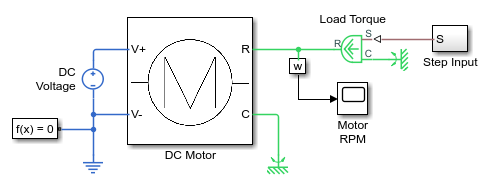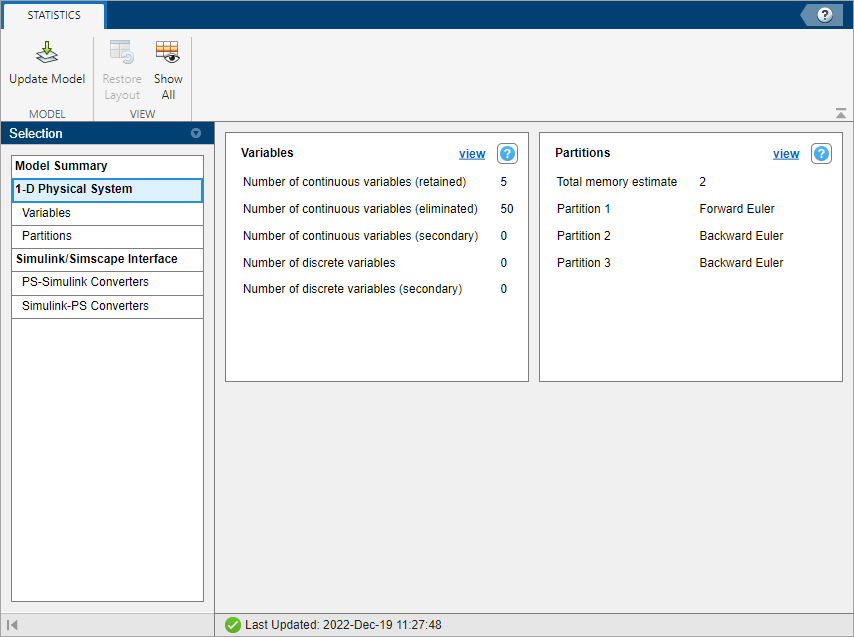## Model Statistics Available when Using the Partitioning Solver

If your model uses a Partitioning local solver, the Statistics Viewer contains additional statistics specific to this solver type:

• Number of partitions — This statistic represents the total number of partitions in the model. When you expand this node, you can view additional statistics, listed below.

• Total memory estimate — This statistic represents the estimate for memory usage, in kB, for this model when using the exhaustive partition storage method.

• Additional nodes, named Partition1, Partition2, and so on, up to the total number of partitions in the model. For each of these nodes, the Value column lists the integration method applied to this partition. Possible methods are forward Euler (explicit) and backward Euler (implicit). For each partition node, you can also see:

• Equation type — The Value column for this statistic lists the type of the equations in the partition. Possible types are linear time-invariant, switched linear, and linear time-varying.

• Number of variables — This statistic represents the number of scalar variables in the partition. When you select this node, the Sources section of the Statistics Viewer lists all the variables that fall under this statistic. For each variable, the Source column contains the full path to the variable, starting from the top-level model, with a link to the relevant block. If you click the link in the Source column, the corresponding block is highlighted in the block diagram. The Value column contains the name of the variable, as it would appear in the Initial Targets section of the block dialog box.

• Number of equations — This statistic represents the number of scalar equations in the partition. Sources of the equations are provided, if they are available. When you select this node, the Sources section of the Statistics Viewer lists all the blocks that provide the equations. If the block source code is available (that is, not protected), clicking the link in the Source column opens the Simscape™ source file for this block in the MATLAB® Editor, pointing to the appropriate equation.

• Number of modes — If the Equation type is linear time-invariant or switched linear, this statistic represents the number of modes in the partition. Every `if` and `elseif` statement in Simscape source code corresponds to a mode. In general, when an implicit integration method is applied to a partition, the more modes there are, the more iterations are possibly needed to solve the equations for this partition.

• Number of configurations — This statistic represents the total number of different systems of linear equations that need to be solved when simulating the partition. In the linear time-invariant or switched linear cases, this is 2^n, where n is the number of modes in the partition. To accelerate computation, decompositions of some systems are cached for each set of modes (the Partition storage method parameter in the Solver Configuration block defines how the decompositions are cached). If this number exceeds the greatest possible supported unsigned integer value, the Value column for this statistic displays `Overflow`.

• Memory estimate — This statistic represents the estimate for memory usage, in kB, for this partition when using the exhaustive partition storage method.

### Estimate the Memory Budget for Exhaustive Partitioning Storage

This example shows how you can use the Statistics Viewer to estimate the memory budget needed for simulating a model that uses the Partitioning solver.

1. Open the Permanent Magnet DC Motor example model.2. Double-click the Solver Configuration block, select the Use local solver check box, and then set the Solver type to `Partitioning`.

3. To view model statistics, in the model window, on the Debug tab, click Simscape > Statistics Viewer. Click the button in the toolbar of the viewer window, if necessary, to populate the viewer with data.

4. Expand the Number of partitions node.The Total memory estimate statistic indicates that the estimate for memory usage for this model is 3 kB. When you use the exhaustive partition storage method, the default memory budget allocated for partition storage is 1024 kB. Therefore, the default memory budget value is sufficient for simulating this model, and you can even reduce it, if necessary.

If the memory estimate is unexpectedly large, you can investigate further by expanding each of the individual partition nodes and checking the Memory estimate value for that partition.